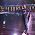## Friday, August 30, 2013

### Moving Ants in Triangluar spiral - Classic Puzzle

There are three ants.

They are sitting on the three corners of an equilateral triangle.

If each ant randomly picks a direction. And start moving along the edge.

What is the probability that no two ants collide?

1.2.@Rahul Lamba - Can you explain How the answer is 1/6.

I thought as each ant has 2 directions independent of other ant's decision so total paths are 2x2x2=8 and ants will not collide iff all move towards single direction (clockwise or anticlock wise) So 2 ways. So probablity is 2/8 = 1/4.

May be I am wrong or missing something here.
Can you explain please.

3.Thankyou JobPuzzles.com
I got same puzzle in my interview